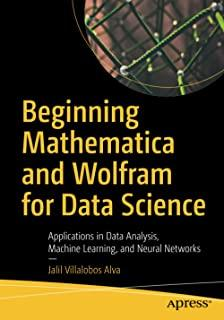### Beginning Mathematica and Wolfram for Data Science: Applications in Data Analysis, Machine Learning, and Neural Networks### Huge savings for students

Each student receives a 50% discount off of most books in the HSG Book Store. During class, please ask the instructor about purchase details.
 List Price: \$44.99 Price: \$22.50 You Save: \$22.50
8

Enhance your data science programming and analysis with the Wolfram programming language and Mathematica, an applied mathematical tools suite. The book will introduce you to the Wolfram programming language and its syntax, as well as the structure of Mathematica and its advantages and disadvantages.

You'll see how to use the Wolfram language for data science from a theoretical and practical perspective. Learning this language makes your data science code better because it is very intuitive and comes with pre-existing functions that can provide a welcoming experience for those who use other programming languages.

You'll cover how to use Mathematica where data management and mathematical computations are needed. Along the way you'll appreciate how Mathematica provides a complete integrated platform: it has a mixed syntax as a result of its symbolic and numerical calculations allowing it to carry out various processes without superfluous lines of code. You'll learn to use its notebooks as a standard format, which also serves to create detailed reports of the processes carried out.

What You Will Learn

• Use Mathematica to explore data and describe the concepts using Wolfram language commands
• Create datasets, work with data frames, and create tables
• Import, export, analyze, and visualize data
• Work with the Wolfram data repository
• Build reports on the analysis
• Use Mathematica for machine learning, with different algorithms, including linear, multiple, and logistic regression; decision trees; and data clustering

Who This Book Is For

Data scientists new to using Wolfram and Mathematica as a language/tool to program in. Programmers should have some prior programming experience, but can be new to the Wolfram language.

Apress